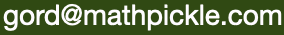Select Page

#### Jumping Frogs

##### (MathPickle, 2017)

Jumping Frogs is a fantastic base to create both casual and complex puzzles. We start at a kindergarten level and work up. After you watch the video, here are other tough problems I got stumped on…

For any initial string of waterlogged (X) and normal lily pads (_), can you always add a finite number of normal lily pads to the end so that it is solvable?

Example… the string _ X X X can be solved by adding four normal lily pads to the end:
_ X X X _ _ _ _.

Example… the string _ X _ X _ _ _ X X can be solved by adding six normal lily pads to the end:
_ X _ X _ _ _ X X _ _ _ _ _ _.

We can record our results using binary. Normal lily pads are 1 and waterlogged lily pads are 0. So the first example is a binary string 1000 with the answer 10001111. Do all binary strings have answers? If not – what is the lowest binary string that results in a failure.

Solutions for children up to 41

Paper for mathematicians

_____________________

Given n>2 lily pads – the fewest number of waterlogged lily pads that make the frog party impossible is W(n). What is the smallest n for which W(n) = 4?

Example: for n=3 the smallest number lily pads that make it impossible is 1.
_ X _

Example: for n=4 the smallest number lily pads that make it impossible is 2.
_ X _ X
or
_ X X _

Example: for n=14 the smallest number lily pads that make it impossible is 3.
_ X _ X _ _ _ _ _ _ _ _ X _

_____________________

Is W(n) ever more than W(n+1)?

_____________________

How does W(n) behave for large n?

#### Standards for Mathematical Practice

MathPickle puzzle and game designs engage a wide spectrum of student abilities while targeting the following Standards for Mathematical Practice:

##### MP1 Toughen up!

Students develop grit and resiliency in the face of nasty, thorny problems. It is the most sought after skill for our students.

##### MP2 Think abstractly!

Students take problems and reformat them mathematically. This is helpful because mathematics lets them use powerful operations like addition.

##### MP3 Work together!

Students discuss their strategies to collaboratively solve a problem and identify missteps in a failed solution. MathPickle recommends pairing up students for all its puzzles.

##### MP4 Model reality!

Students create a model that mimics the real world. Discoveries made by manipulating the model often hint at something in the real world.

##### MP5 Know the tools.

Students master the tools at their fingertips - whether it's a pencil or an online app.

##### MP6 Be precise!

Students learn to communicate using precise terminology. MathPickle encourages students not only to use the precise terms of others, but to invent and rigorously define their own terms.

##### MP7 Be observant!

Students learn to identify patterns. This is one of the things that the human brain does very well. We sometimes even identify patterns that don't really exist 😉

##### MP8 Be lazy!?!

Students learn to seek for shortcuts. Why would you want to add the numbers one through a hundred if you can find an easier way to do it?

Please use MathPickle in your classrooms. If you have improvements to make, please contact me. I'll give you credit and kudos 😉 For a free poster of MathPickle's ideas on elementary math education go here.

## Gordon Hamilton

##### (MMath, PhD)